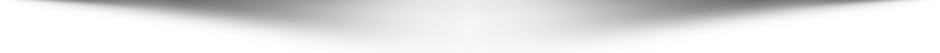# Test Your Knowledge of Chemistry with Our Quiz

Welcome to our Chemistry Quiz! Test your knowledge on the fundamental principles and concepts of chemistry with these 30 questions. From atoms to chemical reactions and everything in between, this quiz covers it all. Don't forget to read the questions carefully and choose the correct answer from the four options provided. Good luck!

Simple Everyday Personal Finance Tips for Everyone - Free

## Chemistry Quiz## All Chemistry Quiz Questions

1. What is the chemical symbol for gold?
2. What is the chemical symbol for lead?
3. Which gas is most commonly found in the Earth's atmosphere?
4. What is the formula for water?
5. What is the process of converting a solid into a gas called?
6. Which compound is produced when an acid reacts with a base?
7. What is the pH value of a neutral solution?
8. Which element is found in all organic compounds?
9. What is the process of burning called?
10. What is the chemical symbol for helium?
11. What is the chemical symbol for sodium?
12. Which element is the most abundant in the Earth's crust?
13. What is the name of the process where a metal reacts with oxygen to form a metal oxide?
14. What is the name of the process where a liquid turns into a gas at a temperature below its boiling point?
15. Which gas is known as laughing gas?
16. What is the name of the bond where two atoms share electrons?
17. What is the name of the process where a gas turns into a liquid?
18. Which element is found in all acids?
19. Which of the following elements is a metalloid?
20. What is the process called when a solid turns into a gas without first becoming a liquid?
21. Which of the following is a noble gas?
22. What is the most common element in the Earth's atmosphere?
23. Which of the following is not a type of chemical reaction?
24. What is the chemical formula for table salt?
25. What is the chemical symbol for iron?
26. What type of chemical reaction involves the combination of two or more substances to form a new compound?
27. What is the state of matter of a substance that has a definite shape and volume?
28. Which of the following is a common acid found in citrus fruits?
29. Which of the following is a strong acid?
30. Which of the following is a weak acid?